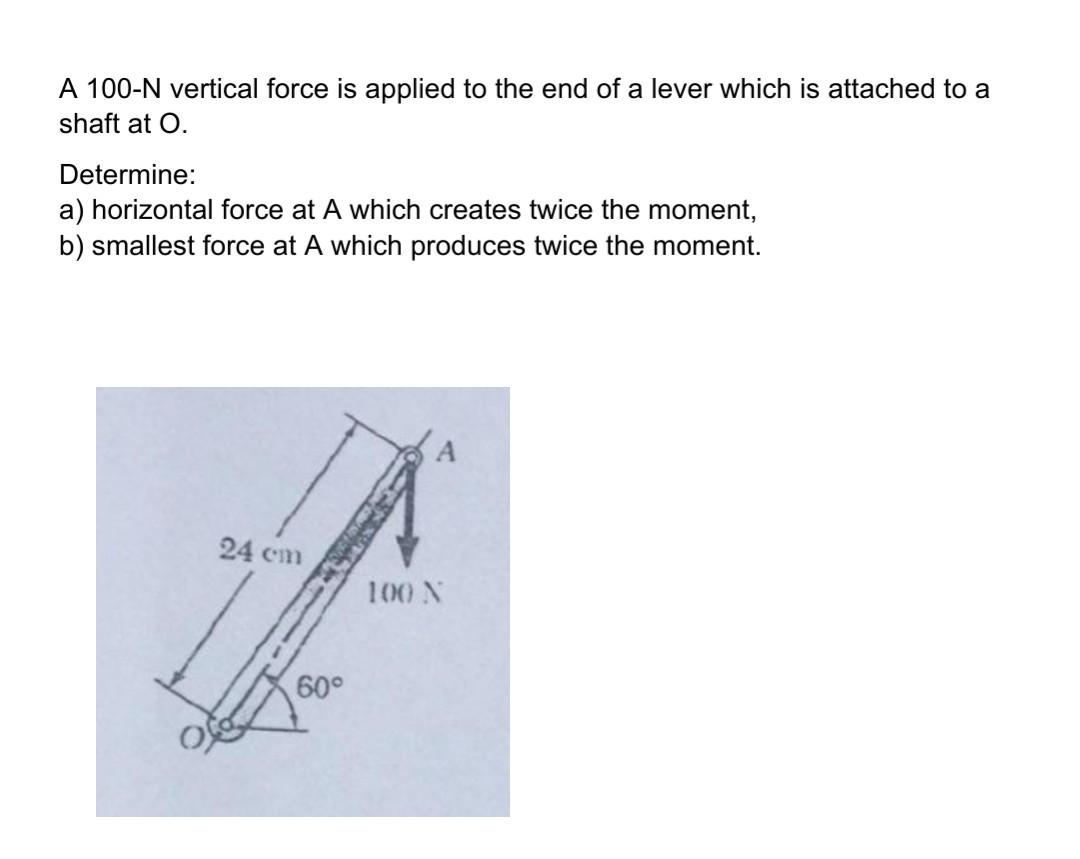# A 100-N vertical force is applied to the end of a lever which is attached to a shaft at 0. Determine: a) horizontal force at A which creates twice the moment, b) smallest force at A which produces twice the moment.

Question-AnswerCategory: Engineering MechanicsA 100-N vertical force is applied to the end of a lever which is attached to a shaft at 0. Determine: a) horizontal force at A which creates twice the moment, b) smallest force at A which produces twice the moment.A 100-N vertical force is applied to the end of a lever which is attached to a shaft at 0. Determine: a) horizontal force at A which creates twice the moment, b) smallest force at A which produces twice the moment.